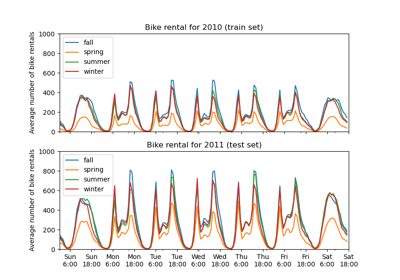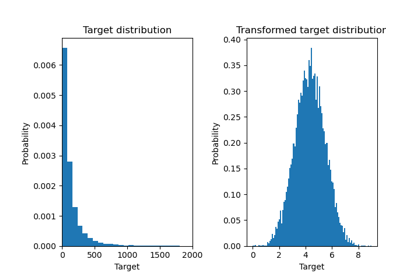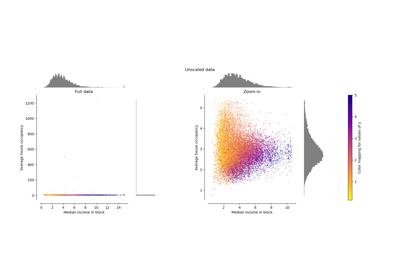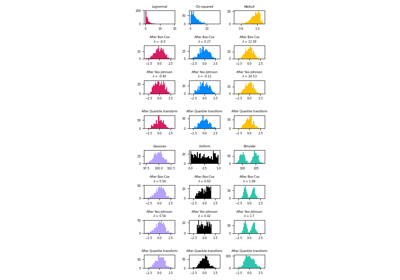# sklearn.preprocessing.QuantileTransformer¶

class sklearn.preprocessing.QuantileTransformer(*, n_quantiles=1000, output_distribution='uniform', ignore_implicit_zeros=False, subsample=10000, random_state=None, copy=True)[source]

Transform features using quantiles information.

This method transforms the features to follow a uniform or a normal distribution. Therefore, for a given feature, this transformation tends to spread out the most frequent values. It also reduces the impact of (marginal) outliers: this is therefore a robust preprocessing scheme.

The transformation is applied on each feature independently. First an estimate of the cumulative distribution function of a feature is used to map the original values to a uniform distribution. The obtained values are then mapped to the desired output distribution using the associated quantile function. Features values of new/unseen data that fall below or above the fitted range will be mapped to the bounds of the output distribution. Note that this transform is non-linear. It may distort linear correlations between variables measured at the same scale but renders variables measured at different scales more directly comparable.

Read more in the User Guide.

New in version 0.19.

Parameters:
n_quantilesint, default=1000 or n_samples

Number of quantiles to be computed. It corresponds to the number of landmarks used to discretize the cumulative distribution function. If n_quantiles is larger than the number of samples, n_quantiles is set to the number of samples as a larger number of quantiles does not give a better approximation of the cumulative distribution function estimator.

output_distribution{‘uniform’, ‘normal’}, default=’uniform’

Marginal distribution for the transformed data. The choices are ‘uniform’ (default) or ‘normal’.

ignore_implicit_zerosbool, default=False

Only applies to sparse matrices. If True, the sparse entries of the matrix are discarded to compute the quantile statistics. If False, these entries are treated as zeros.

subsampleint, default=10_000

Maximum number of samples used to estimate the quantiles for computational efficiency. Note that the subsampling procedure may differ for value-identical sparse and dense matrices.

random_stateint, RandomState instance or None, default=None

Determines random number generation for subsampling and smoothing noise. Please see subsample for more details. Pass an int for reproducible results across multiple function calls. See Glossary.

copybool, default=True

Set to False to perform inplace transformation and avoid a copy (if the input is already a numpy array).

Attributes:
n_quantiles_int

The actual number of quantiles used to discretize the cumulative distribution function.

quantiles_ndarray of shape (n_quantiles, n_features)

The values corresponding the quantiles of reference.

references_ndarray of shape (n_quantiles, )

Quantiles of references.

n_features_in_int

Number of features seen during fit.

New in version 0.24.

feature_names_in_ndarray of shape (n_features_in_,)

Names of features seen during fit. Defined only when X has feature names that are all strings.

New in version 1.0.

quantile_transform

Equivalent function without the estimator API.

PowerTransformer

Perform mapping to a normal distribution using a power transform.

StandardScaler

Perform standardization that is faster, but less robust to outliers.

RobustScaler

Perform robust standardization that removes the influence of outliers but does not put outliers and inliers on the same scale.

Notes

NaNs are treated as missing values: disregarded in fit, and maintained in transform.

For a comparison of the different scalers, transformers, and normalizers, see examples/preprocessing/plot_all_scaling.py.

Examples

>>> import numpy as np
>>> from sklearn.preprocessing import QuantileTransformer
>>> rng = np.random.RandomState(0)
>>> X = np.sort(rng.normal(loc=0.5, scale=0.25, size=(25, 1)), axis=0)
>>> qt = QuantileTransformer(n_quantiles=10, random_state=0)
>>> qt.fit_transform(X)
array([...])


Methods

 fit(X[, y]) Compute the quantiles used for transforming. fit_transform(X[, y]) Fit to data, then transform it. get_feature_names_out([input_features]) Get output feature names for transformation. get_params([deep]) Get parameters for this estimator. Back-projection to the original space. set_output(*[, transform]) Set output container. set_params(**params) Set the parameters of this estimator. Feature-wise transformation of the data.
fit(X, y=None)[source]

Compute the quantiles used for transforming.

Parameters:
X{array-like, sparse matrix} of shape (n_samples, n_features)

The data used to scale along the features axis. If a sparse matrix is provided, it will be converted into a sparse csc_matrix. Additionally, the sparse matrix needs to be nonnegative if ignore_implicit_zeros is False.

yNone

Ignored.

Returns:
selfobject

Fitted transformer.

fit_transform(X, y=None, **fit_params)[source]

Fit to data, then transform it.

Fits transformer to X and y with optional parameters fit_params and returns a transformed version of X.

Parameters:
Xarray-like of shape (n_samples, n_features)

Input samples.

yarray-like of shape (n_samples,) or (n_samples, n_outputs), default=None

Target values (None for unsupervised transformations).

**fit_paramsdict

Returns:
X_newndarray array of shape (n_samples, n_features_new)

Transformed array.

get_feature_names_out(input_features=None)[source]

Get output feature names for transformation.

Parameters:
input_featuresarray-like of str or None, default=None

Input features.

• If input_features is None, then feature_names_in_ is used as feature names in. If feature_names_in_ is not defined, then the following input feature names are generated: ["x0", "x1", ..., "x(n_features_in_ - 1)"].

• If input_features is an array-like, then input_features must match feature_names_in_ if feature_names_in_ is defined.

Returns:
feature_names_outndarray of str objects

Same as input features.

get_params(deep=True)[source]

Get parameters for this estimator.

Parameters:
deepbool, default=True

If True, will return the parameters for this estimator and contained subobjects that are estimators.

Returns:
paramsdict

Parameter names mapped to their values.

inverse_transform(X)[source]

Back-projection to the original space.

Parameters:
X{array-like, sparse matrix} of shape (n_samples, n_features)

The data used to scale along the features axis. If a sparse matrix is provided, it will be converted into a sparse csc_matrix. Additionally, the sparse matrix needs to be nonnegative if ignore_implicit_zeros is False.

Returns:
Xt{ndarray, sparse matrix} of (n_samples, n_features)

The projected data.

set_output(*, transform=None)[source]

Set output container.

See Introducing the set_output API for an example on how to use the API.

Parameters:
transform{“default”, “pandas”}, default=None

Configure output of transform and fit_transform.

• "default": Default output format of a transformer

• "pandas": DataFrame output

• None: Transform configuration is unchanged

Returns:
selfestimator instance

Estimator instance.

set_params(**params)[source]

Set the parameters of this estimator.

The method works on simple estimators as well as on nested objects (such as Pipeline). The latter have parameters of the form <component>__<parameter> so that it’s possible to update each component of a nested object.

Parameters:
**paramsdict

Estimator parameters.

Returns:
selfestimator instance

Estimator instance.

transform(X)[source]

Feature-wise transformation of the data.

Parameters:
X{array-like, sparse matrix} of shape (n_samples, n_features)

The data used to scale along the features axis. If a sparse matrix is provided, it will be converted into a sparse csc_matrix. Additionally, the sparse matrix needs to be nonnegative if ignore_implicit_zeros is False.

Returns:
Xt{ndarray, sparse matrix} of shape (n_samples, n_features)

The projected data.

## Examples using sklearn.preprocessing.QuantileTransformer¶Partial Dependence and Individual Conditional Expectation Plots

Partial Dependence and Individual Conditional Expectation PlotsEffect of transforming the targets in regression model

Effect of transforming the targets in regression modelCompare the effect of different scalers on data with outliers

Compare the effect of different scalers on data with outliersMap data to a normal distribution

Map data to a normal distribution